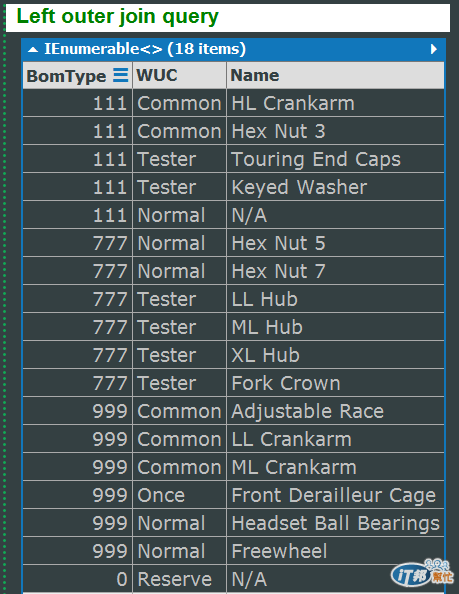#DAY 30
2

## LINQ自學筆記-語法應用-聚合資料-DefaultIfEmpty 運算子、實做 Left Outer Join 效果

Join 運算子的第四篇文章，將和大家分享如何利用 GroupJoin 運算子完成 Left outer join 的效果。

``````public static IEnumerable<TSource> DefaultIfEmpty<TSource>(
this IEnumerable<TSource> source
)
public static IEnumerable<TSource> DefaultIfEmpty<TSource>(
this IEnumerable<TSource> source,
TSource defaultValue
)
``````

DefaultIfEmpty 運算子是 IEnumerable<TSource> 的擴充方法，呼叫它時，會回傳 IEnumerable<TSource>，來源和結果看起來都一樣，唯一的差異是 DefaultIfEmpty 運算子所回傳的序列，其中若有項目是 null，則會以該項目型別（TSource）的預設值帶入，或者我們可以利用第二個多載方法自訂項目為 null 時的預設值。

But，是的，人生最機車的 But 又出現了！.Net 3.5 的 LINQ to SQL/Entities 完全不支援 DefaultIfEmpty 運算子，.Net 4.0/4.5 的 LINQ to SQL 只支援第一個無參數的多載方法，至於 LINQ to Entities ，MSDN 文件上是寫兩個多載方法都支援，不過實際在 .Net 4.0 上，使用第二個多載方法還是會出現 NotSupportedException 例外，原因我還沒查出來，有結果再回補文章，總之，使用時請留意，才不會老是發生 NotSupportedException 錯誤。

``````void Main()
{
var BOMTable = DataProvider.getBOM();
var Equips = DataProvider.getEquips();
var query =
from b in BOMTable
join e in Equips
on new { b.BomType, b.WUC } equals new { e.BomType, e.WUC } into be
from obj in be.DefaultIfEmpty()
select new
{
b.BomType, b.WUC,
Name = obj == null ? "N/A" : obj.Name
};
query.Dump("Left outer join query");
}
````````````BOMTable
.GroupJoin (
Equips,
b =>
new
{
BomType = b.BomType,
WUC = b.WUC
},
e =>
new
{
BomType = e.BomType,
WUC = e.WUC
},
(b, be) =>
new
{
b = b,
be = be
}
)
.SelectMany (
temp0 => temp0.be.DefaultIfEmpty (),
(temp0, obj) =>
new
{
BomType = temp0.b.BomType,
WUC = temp0.b.WUC,
Name = (obj == null) ? "N/A" : obj.Name
}
)
``````

``````SELECT
[Extent1].[BomType] AS [BomType],
[Extent1].[WUC] AS [WUC],
CASE WHEN ([Extent2].[Name] IS NULL) THEN N'N/A' ELSE [Extent2].[Name] END AS [C1]
FROM  [dbo].[BOMTable] AS [Extent1]
LEFT OUTER JOIN [dbo].[Equip] AS [Extent2] ON ([Extent1].[BomType] = [Extent2].[BomType]) AND ([Extent1].[WUC] = [Extent2].[WUC])
``````

``````void Main()
{
var BOMTable = DataProvider.getBOM();
var Equips = DataProvider.getEquips();
var query =
from b in BOMTable
join e in Equips
on new { b.BomType, b.WUC } equals new { e.BomType, e.WUC } into be
from obj in be.DefaultIfEmpty(new Equip {Name = default(string)})
select new
{
b.BomType, b.WUC,
Name = obj.Name ?? "N/A"
};
query.Dump("Left outer join query");
}
``````

``````BOMTable
.GroupJoin (
Equips,
b =>
new
{
BomType = b.BomType,
WUC = b.WUC
},
e =>
new
{
BomType = e.BomType,
WUC = e.WUC
},
(b, be) =>
new
{
b = b,
be = be
}
)
.SelectMany (
temp0 =>
temp0.be
.DefaultIfEmpty (
new Equip()
{
Name = null
}
),
(temp0, obj) =>
new
{
BomType = temp0.b.BomType,
WUC = temp0.b.WUC,
Name = (obj.Name ?? "N/A")
}
)
``````

``````void Main()
{
var BOMTable = DataProvider.getBOM();
var Equips = DataProvider.getEquips();
var query =
from b in BOMTable
join e in Equips
on new { b.BomType, b.WUC } equals new { e.BomType, e.WUC } into be
select be.DefaultIfEmpty(new Equip
{
BomType = b.BomType,
WUC = b.WUC,
Name = "N/A"
});
query.SelectMany(q => q).Dump("Left outer join query");
}
``````

0

0